# Planar and Nonplanar Tetrarhons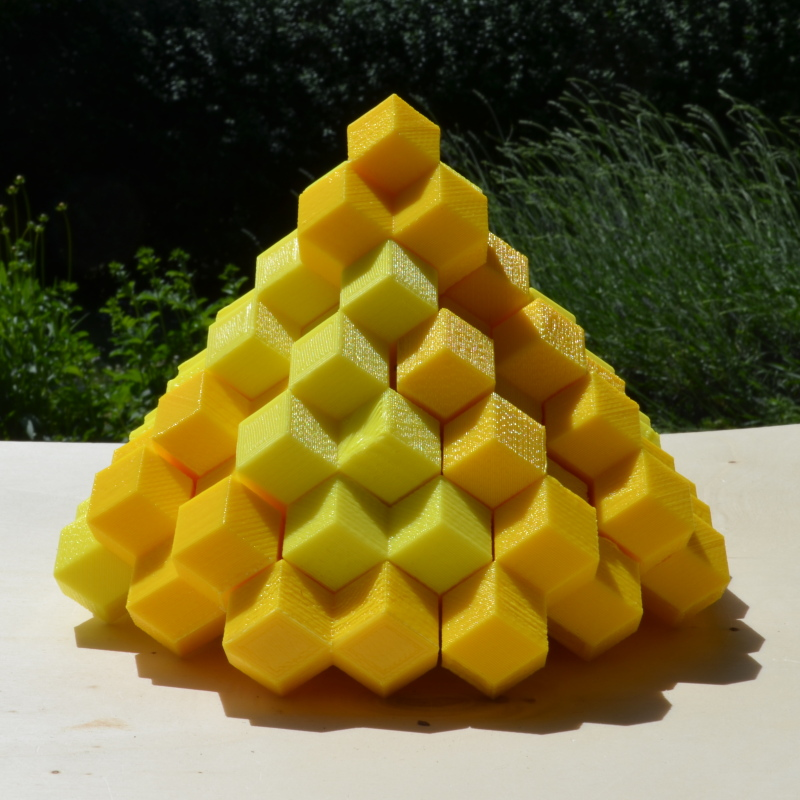This is the complete set of all 28 tetrarhons with a total number of 28*4 = 112 rhombic dodecahedrons. Nonplanar pieces are red colored.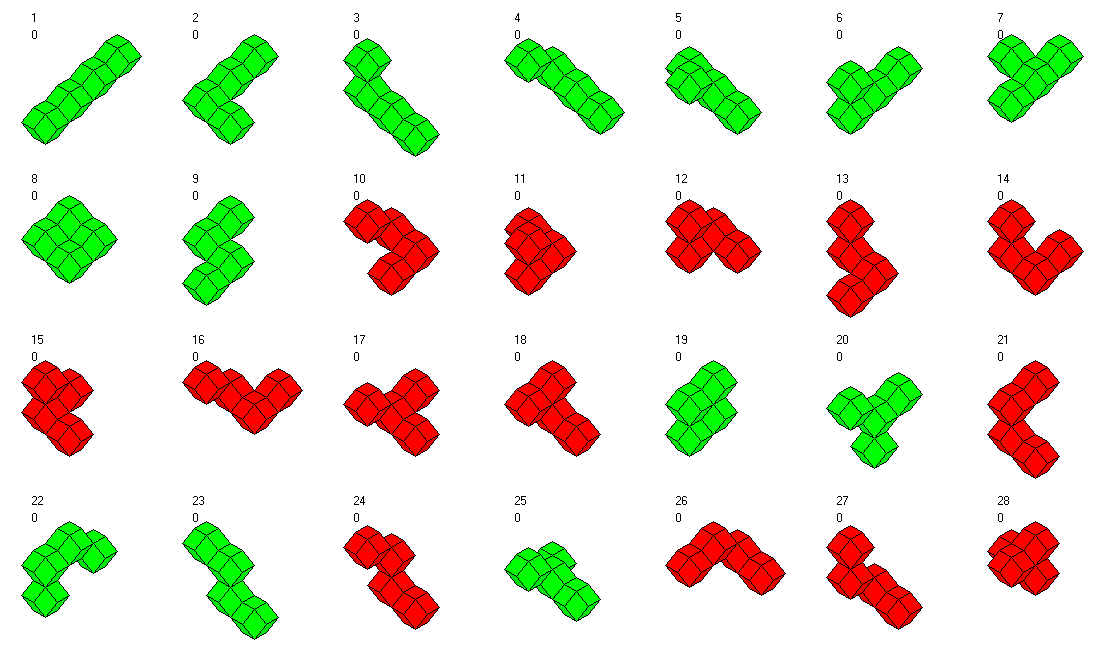A square pyramid of size 7 consists of 140 units, too many for the set. But we can remove 4*7=28 units at the four edges getting a figure, which is rotationally symmetric with respect to the z-axis. It's shown above To see the layers of this construction and the following ones click the pictures.

Removing other units from the square pyramid delivers another rotationally symmetric figure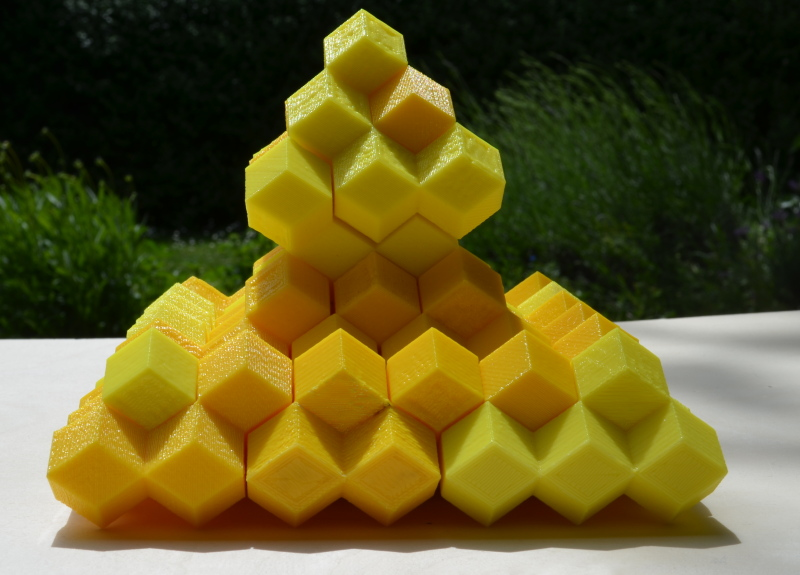Using constructions for the planar and the nonplanar tetrarhons yields a ring, which is also rotationally symmetric but not mirror symmetric.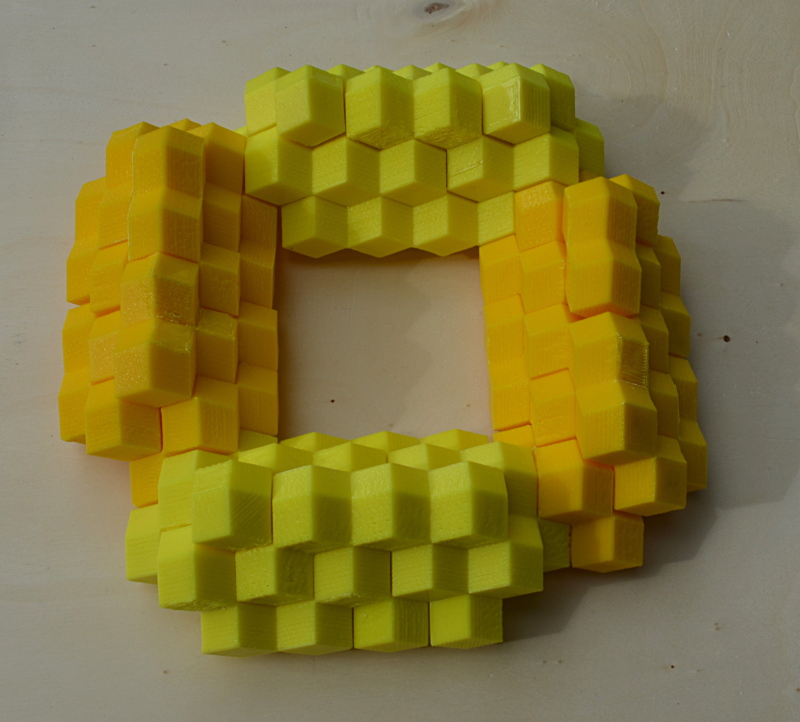Square pyramids of size 5 have a volume of 55 units. If we combine two rhombic dodecahedrons with two pyramids it's just enough for the whole set.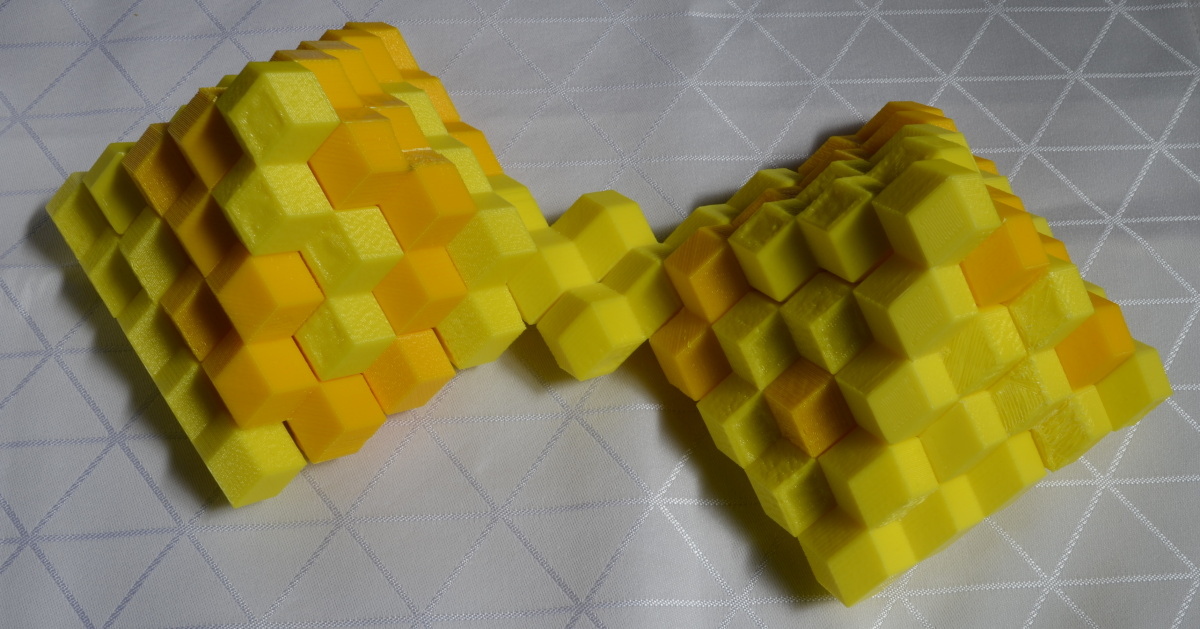The 6x7 roof is another figure with a square grid at the bottom.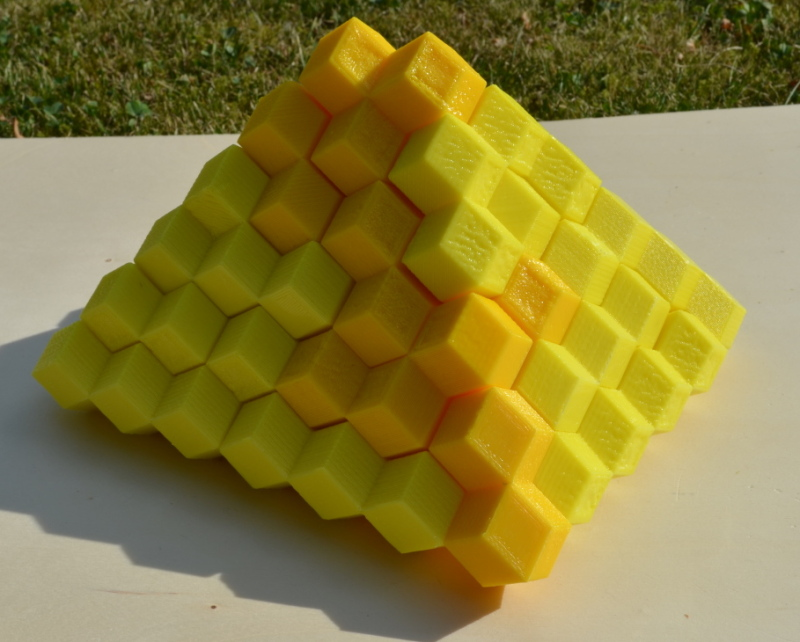For the hexagonal grid at the bottom I found a combination of a size 7 tetrahedron and a size 7 flat triangle.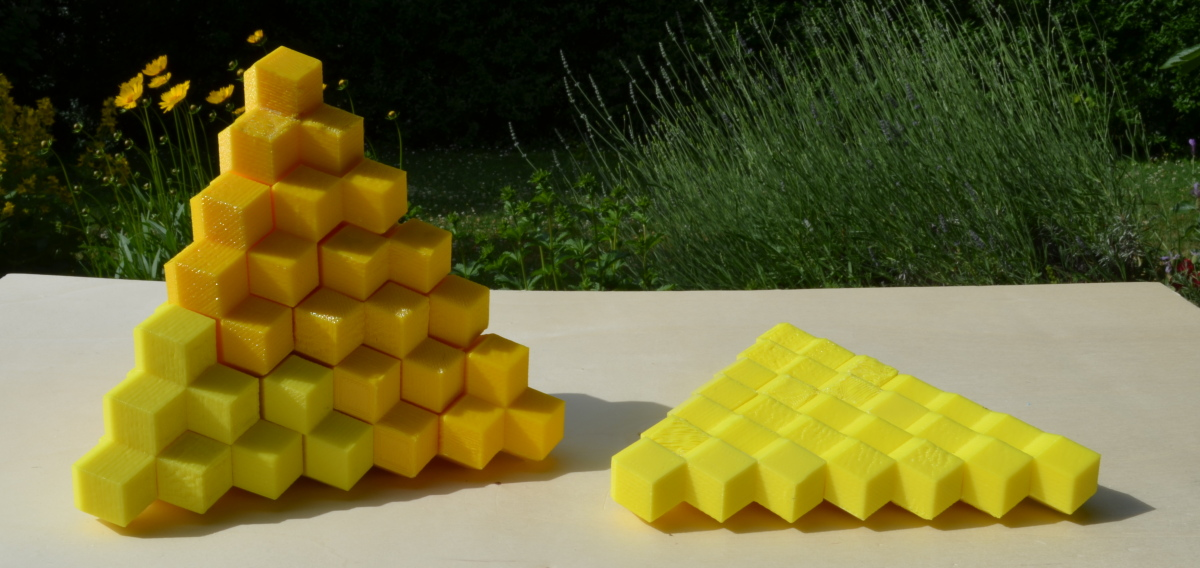What about kind of boxes? I looked for three options to stack 2n-1 layers of alternating size.
• (a x b)*n + (a+1)x(b+1)*(n-1)=112, => n=5, a=1, b=8, a,b interchangeable
• (a x b)*n + (a-1)x(b-1)*(n-1)=112, => no solution
• (a x b)*n + (a-1)x(b+1)*(n-1)=112, => n=9, a=2, b=4 or n=2, a=9, b=4

In the calculation it's assumed that the corners with four edges are on the ground. For stable physical constructions the boxes were turned to get the rhombs on the ground. The boxes on the right are different although 4x9 rhombs are at the top. In the upper picture we have chains of 9 rhombs and in the lower picture we have chains of four rhombs.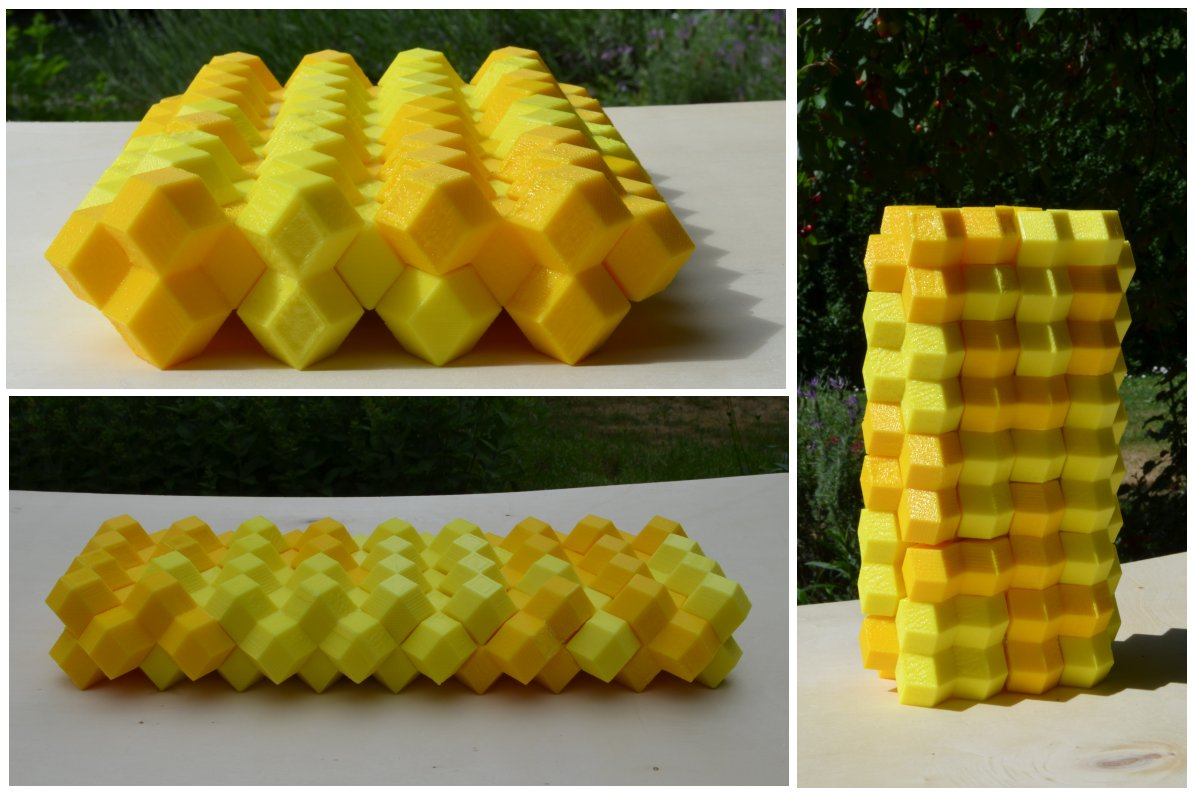Back
Home